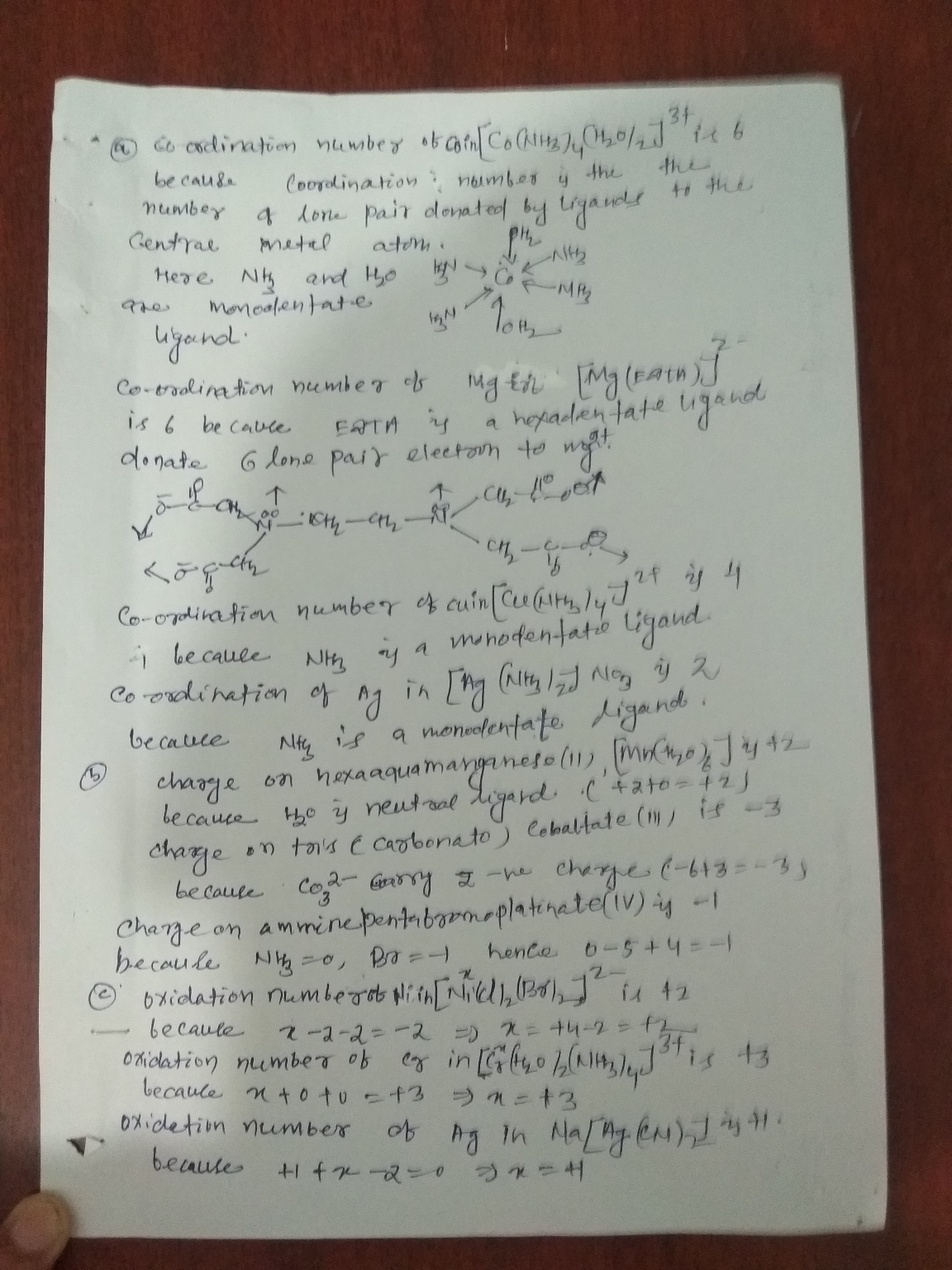In: Chemistry

# What is the coordination number for each of the following complexes or compounds? [Co(NH3)4(H2O)2]3+[Co(NH3)4(H2O)2]3+ [Mg(EDTA)]2−[Mg(EDTA)]2− [Cu(NH3)4]2+[Cu(NH3)4]2+...

What is the coordination number for each of the following complexes or compounds?

1. [Co(NH3)4(H2O)2]3+[Co(NH3)4(H2O)2]3+
2. [Mg(EDTA)]2−[Mg(EDTA)]2−
3. [Cu(NH3)4]2+[Cu(NH3)4]2+
4. [Ag(NH3)2]NO3[Ag(NH3)2]NO3

Enter the coordination numbers, in the order that the complexes are listed, as four integers separated by commas (e.g., 1,2,3,4).

View Available Hint(s)

Submit

Part B

What is the charge on each of the following complex ions?

1. hexaaquamanganese(II), [Mn(H2O)6]?[Mn(H2O)6]?
2. tris(carbonato)cobaltate(III), [Co(CO3)3]?[Co(CO3)3]?
3. amminepentabromoplatinate(IV), [Pt(NH3)Br5]?[Pt(NH3)Br5]?

Express the charges numerically, in the order that the complexes are listed, separated by commas. For a nonzero charge, be sure to include the sign (e.g., +1,-2,+3).

View Available Hint(s)

Submit

Part C

What is the oxidation number of the central metal ion in each of the following complexes or compounds?

1. [NiCl2Br2]2−[NiCl2Br2]2−
2. [Cr(H2O)2(NH3)4]3+[Cr(H2O)2(NH3)4]3+
3. Na[Ag(CN)2]Na[Ag(CN)2]

Express the oxidation numbers numerically, in the order that the complexes are listed, separated by commas. For a nonzero oxidation number, be sure to include the sign (e.g., +1,-2,+3).

View Available Hint(s)

## Solutions

##### Expert Solution## Related Solutions

##### 1. What is the coordination number for each of the following complexes? [Co(NH3)4Cl2]+ [Mg(EDTA)]2? [Zn(H2O)4]2+ Na[Au(CN)2]...
1. What is the coordination number for each of the following complexes? [Co(NH3)4Cl2]+ [Mg(EDTA)]2? [Zn(H2O)4]2+ Na[Au(CN)2] Enter the coordination numbers, in the order that the complexes are listed, as four integers separated by commas (e.g., 1,2,3,4). 2. What is the charge on each of the following complex ions? hexaaquachromium(II), [Cr(H2O)6]? tris(carbonato)aluminate(III), [Al(CO3)3]? amminepentabromoplatinate(IV), [Pt(NH3)Br5]? Express the charges numerically, in the order that the complexes are listed, separated by commas. For a nonzero charge, be sure to include the sign (e.g.,...
##### 1.What is the coordination number for each of the following complexes? [Co(NH3)4Cl2]+ [Pb(EDTA)]2? [Cu(NH3)4]2+ [Ag(NH3)2]NO3 2.What...
1.What is the coordination number for each of the following complexes? [Co(NH3)4Cl2]+ [Pb(EDTA)]2? [Cu(NH3)4]2+ [Ag(NH3)2]NO3 2.What is the charge on each of the following complex ions? hexaaquachromium(II), [Cr(H2O)6]? tris(carbonato)ferrate(III), [Fe(CO3)3]? diaquatetrabromovanadate(III), [V(H2O)2Br4]? 3.What is the oxidation number of the central metal ion in each of the following complexes? [NiCl2Br2]2? [Fe(H2O)2(NH3)4]3+ Na[Rh(CN)2]
##### What is the formal name for each of the following coordination compounds? a. [Co(H2O)3(NH3)3](NO3)3 b. Na3[FeF6]...
What is the formal name for each of the following coordination compounds? a. [Co(H2O)3(NH3)3](NO3)3 b. Na3[FeF6] c. [Mn(en)2Br2]Cl2 d. Ba[Sn(OH)4]
##### Classify each of the following coordination compounds according to the coordination number. [CuCl2]-, [AlCl4]-, [Cr(CO)6], [Ag(NH3)2]+,...
Classify each of the following coordination compounds according to the coordination number. [CuCl2]-, [AlCl4]-, [Cr(CO)6], [Ag(NH3)2]+, [HgI3]-, Ba[FaBr4]2, K3[CoF6], [Fe(CO)5] The oxidation state is for the central metal atom in [Ni(CN)5]3–.
##### Name the following complexes (Coordination Chemistry): PtCl42-, Hg(CN)42-, Ni(NH3)62+, Fe(PO4)23-, Co(NH3)5(H2O)3+, CaHCO3+
Name the following complexes (Coordination Chemistry): PtCl42-, Hg(CN)42-, Ni(NH3)62+, Fe(PO4)23-, Co(NH3)5(H2O)3+, CaHCO3+
##### Name the complex ion [Cu(NH3)2(H2O)4]2+. The oxidation number of copper is +2.
Name the complex ion [Cu(NH3)2(H2O)4]2+. The oxidation number of copper is +2.
##### Calculate the equilibrium concentrations of NH3, Cu2+, [Cu(NH3)]2+, [Cu(NH3)2]2+, [Cu(NH3)3]2+, and [Cu(NH3)4]2+ in a solution made...
Calculate the equilibrium concentrations of NH3, Cu2+, [Cu(NH3)]2+, [Cu(NH3)2]2+, [Cu(NH3)3]2+, and [Cu(NH3)4]2+ in a solution made by mixing 500.0 mL of 3.00 M NH3 with 500.0 mL of 2.00 x 10-3 M Cu(NO3)2. The sequential equilibria are Cu2+ (aq) + NH3(aq) ⇐⇒ [Cu(NH3)]2+ (aq) K1 = 1.86 x 104 [Cu(NH3)]2+ (aq) + NH3(aq) ⇐⇒ [Cu(NH3)2]2+ (aq) K2 = 3.88 x 103 [Cu(NH3)2]2+ (aq) + NH3(aq) ⇐⇒ [Cu(NH3)3]2+ (aq) K3 = 1.00 x 103 [Cu(NH3)3]2+ (aq) + NH3(aq) ⇐⇒ [Cu(NH3)4]2+ (aq)...
##### PART I - Synthesis and purification of [Cu(NH3)4]SO4.H2O Compound Mass (g) CuSO4.H2O 5.090 g [Cu(NH3)4]SO4.H2O 1.880...
PART I - Synthesis and purification of [Cu(NH3)4]SO4.H2O Compound Mass (g) CuSO4.H2O 5.090 g [Cu(NH3)4]SO4.H2O 1.880 g 1. limiting reagent determination (use the stoichiometric ratio to determine the limiting reactant) CuSO4.5H2O (s) + 4NH3 (aq) → [Cu(NH3)4]SO4.H2O (aq) + H2O (l) 2. percentage yield of crude product (remember to include the 0.025 g of compound you take for determining the absorption spectrum). PART II - Spectrophotometric analysis of the coordination complex Compound Quantity Complex mass (g) 0.026 g Volume of...
##### What is the systematic name of [Os(NH3)2(en)2]Cl3, [Co(NH3)3(en)Cl]NO3, and (NH4)2[Fe(EDTA)]?
What is the systematic name of [Os(NH3)2(en)2]Cl3, [Co(NH3)3(en)Cl]NO3, and (NH4)2[Fe(EDTA)]?
##### Cu in [Cu(CN)4]2- and [Cu(NH3)4]2+ has d9 electron configuration ,so the geometry should be tetrahedral but...
Cu in [Cu(CN)4]2- and [Cu(NH3)4]2+ has d9 electron configuration ,so the geometry should be tetrahedral but the experiment Show that these two ions have square planar geometry.Please explain this statement.